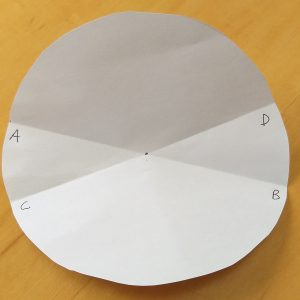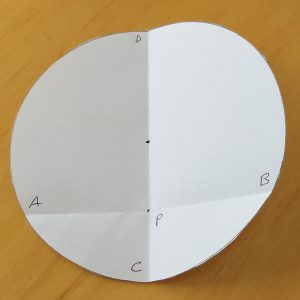Activity 1: Finding the centre of the circle

You will need a cut-out paper circle (about 12cm diameter).

• Use a circle that does not show the centre.
• Fold your circle along a diameter. Mark the ends A and B.
• Now unfold your circle and then fold again along a different diameter and mark the ends C and D. Also mark the point where  AB and CD intersect as O. Why is this the centre?
• Is there another way of finding the centre?Activity 2: Looking at the perpendicular bisector of a chord.

You will need a cut-out paper circle with centre O marked (about 12cm diameter).

• Fold over a segment of the circle to make a chord.  Mark the ends A and B.
• Fold the circle so that A and B come together. Unfold  and mark the points where this line cuts the circle as C and D.
• If AB and CD intersect at P, what do you know about AP and PB? What about angle CPA?
• How is CD related to AB?
• Is CD a diameter of the circle?